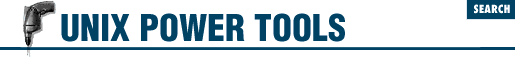home | O'Reilly's CD bookshelfs | FreeBSD | Linux | Cisco | Cisco Exam

#Chapter 9Saving Time on the Command Line## 9.6 String Editing (Colon) Operators

When the C shell and bash do history substitutions ( 11.7 ) they can also edit the substitution. The C shell - but not bash  - can also edit variable substitutions ( 6.8 ) . For instance, in the first example below, when ``` !\$``` contains ``` /a/b/c``` , adding the "head" operator ``` :h``` will give just the head of the pathname, ``` /a/b``` .

For a complete but very terse list of these operators, see the csh manual page. We hope the examples below will help you understand these useful operators.

• ``` :h ``` gives the head of a pathname ( 14.2 ) , as follows:

```% ```

echo /a/b/c
```

/a/b/c
% ```

echo !\$:h
```

echo /a/b
/a/b```

That took off the filename and left the header. This also could be used with C shell variables ( 47.5 ) as:

```% ```

set x = /a/b/c
```

% ```

echo \$x
```

/a/b/c
% ```

echo \$x:h
```

/a/b```

• ``` :r ``` returns the root of a filename:

```% ```

echo xyz.c abc.c
```

xyz.c abc.c
% ```

echo !\$:r
```

echo abc
abc```

The ``` :r``` removed the ``` .c``` from the last argument, leaving the root name. This could also be used in C shell variable names:

```% ```

set x = abc.c
```

% ```

echo \$x:r
```

abc```

• ``` :g ``` For more than one name, you can add the g operator to make the operation global. For example:

 ``` (...) ``` ``` % ``` set x = (a.a b.b c.c) ``` % ``` echo \$x:gr ``` a b c```

The ``` :gr``` operator stripped off all dot (``` .``` ) suffixes. By the way, this use of g does not work with the history commands.

This is the C shell's answer to the basename ( 45.18 ) command.

• ``` :e ``` returns the extension (the part of the name after a dot). Using csh variables:

```% ```

set x=(abc.c)
```

% ```

echo \$x:e
```

c```

No luck using that within history, either.

• ``` :t ``` gives the tail of a pathname - the actual filename without the path:

```% ```

echo /a/b/c
```

/a/b/c
% ```

echo !\$:t
```

c```

With csh variables:

```% ```

set x=(/a/b/c)
```

% ```

echo \$x:t
```

c```

And with multiple pathnames, you can do it globally with:

```% ```

set x=(/a/b/c /d/e/f /g/h/i)
```

% ```

echo \$x:gt
```

c f i```

While the corresponding heads would be:

```% ```

set x=(/a/b/c /d/e/f /g/h/i)
```

% ```

echo \$x:gh
```

/a/b /d/e /g/h```

• ``` :p ``` prints the command, but does not execute it ( 11.10 ) :

```% ```

echo *
```

fn1 fn2 fn3
% ```

!:p
```

echo fn1 fn2 fn3```

• ``` :q ``` prevents further filename expansion, or prints the command as is:

```% ```

echo *
```

fn1 fn2 fn3
% ```

!:q
```

echo *
*```

The first command echoed the files in the directory, and when the ``` :q``` was applied, it echoed only the special character.

• ``` :x ``` is like ``` :q``` , but it breaks the line into words. That is, when using ``` :q``` , it is all one word, while ``` :x``` will break it up into multiple words. [``` :q``` and ``` :x``` are more often used with C shell arrays ( 47.5 ) . - JP  ]

- DR9.5 Build Strings with { }9.7 String Editing in ksh and bash